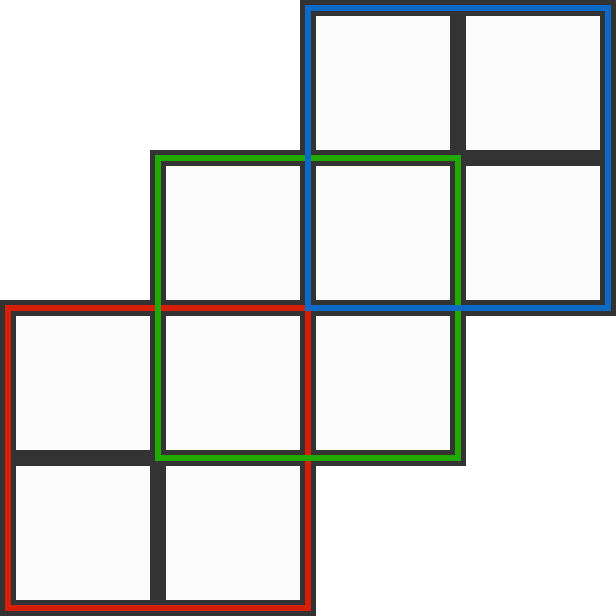# The Maximum Possible Value of P

Algebra Level 1Place the ten integers 2 through 11 into the ten squares such that the sum of the numbers in each $2 \times 2$ square is the same value, $P.$

What is the maximum possible value of $P?$

×